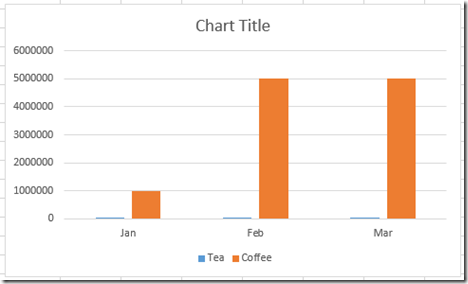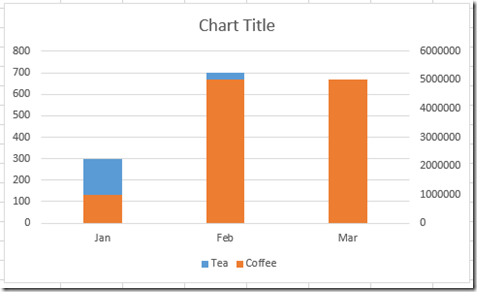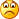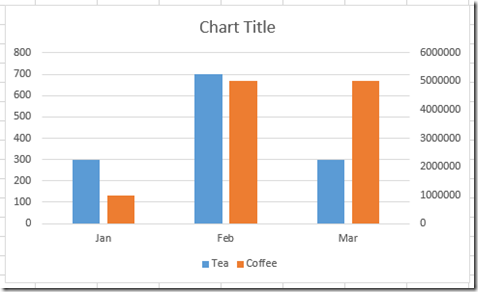# Excel Clustered Column Chart Conundrum

Many, many, many Excel users post this problem in user forums.  And this one always throws me for a loop and takes me time to solve it.  So I thought I would send it out to all the Excel enthusiasts to see if you can fix this Excel Chart.

The Problem:

1) Copy this Data into an Excel Worksheet

A B C
1 Tea Coffee
2 Jan 300 1000000
3 Feb 700 5000000
4 Mar 300 5000000

2) Highlight the Data and Create a 2-D Clustered Column ChartHowever, my data series are not of the same scale, so, I decide to create 2 vertical axis’ so that the scales are distinct for the two data series.  So then I go to the next step.

3) Move the tall orange columns to the secondary axis.OOPS!  This is not the chart I wanted.  Why is my Excel charts now an Excel Stacked Colum Chart?  I just want the two columns in my chart to be separated on 2 different vertical axis.  How can I fix it?

This is the chart I really wanted:How would you make this 2-D Clustered Column Chart with two vertical axis scales?

Copy the data from above and give it a try.  Let me know how you would create this chart in the comments below.  Regardless, comeback tomorrow and see how we can handle this using Excel Charts.

Steve=True